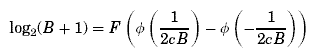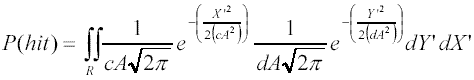CALCULATING IDpr

The probabilistic index of difficulty is calculated with the equation IDpr = F(P(hit)).  The function F is created by substituting different values of B into the left and right sides of the equation:The value of c is determined empirically, but will be somewhere along the lines of 0.07. The following is an Excel spreadsheet demonstrating the function F over various values of B. You can try inserting different values of B to see the corresponding probability and index of difficulty values.

We also need to calculate P(hit). For the 2D case, we use the equation:Where c (values ranges from 0.055 - 0.075) and d (values ranges from 0.025 - 0.035) are determined empirically, A is the distance to the target center, and R is the region created by the target. The probabilities can be calculated using computational methods.  For example, the following Maple script could be used:

maplescript.html

Once a probability is calculated, we can use the F function to obtain the corresponding index of difficulty. The following Excel spreadsheet provides probabilities and their corresponding index of difficulties for various conditions from our second experiment.  We also include the average movement time values:

datasset.xls